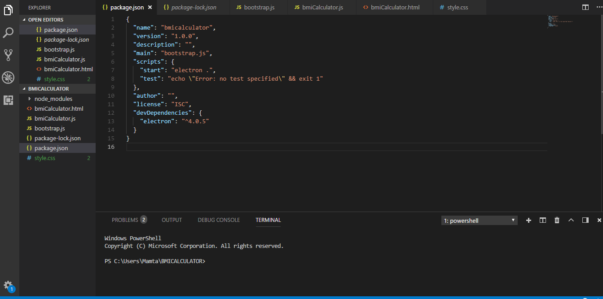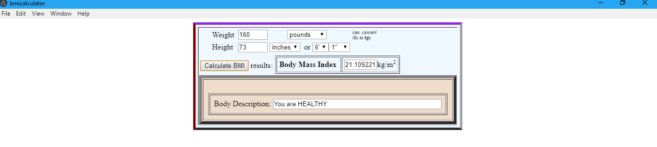Video TutorialsWeb Development TutorialsHow to Create a BMI Calculator Using Electron Framework

# How to Create a BMI Calculator Using Electron Framework

An electron is a framework for creating web applications using HTML, CSS and Javascript. In the Initial phase, the electron was developed for GitHub’s Atom Editor. But gradually, electron got noticed and has since been used to create applications by companies like Microsoft, Facebook, Slack and Docker. Electron combines the best technologies and hence it is platform independent meaning compatible with Mac, Windows and Linux.

### Why Electron?

From the developer’s point of view, they don’t need to worry about writing a specific program for each platform while using this framework. This comes with a host of benefits for both the creator and the user.

Rather than writing applications in traditional programming languages, Electron allows the user to write these applications in 3 main components of many web-pages today:

Meanwhile, if you are interested to learn and create different projects such as sign up form, slideshow, hamburger menu and others using these 3 technologies then you can opt for HTML, CSS and JavaScript Course.

#### Advantages of Electron:

1)Cross-Platform Programs: Since the framework is based on web technologies, software using it is highly compatible with each other. Visual Studio Code(VSC) is an example of an application using this framework.
2)Faster Development Time: Electron allows creating application using languages the web page is made of. So if you know how to write a web page, you will be able to use Electron.
3)Consistency: Applications tend to look and behave the same regardless of the platform on which they are running.

In this blog, we are going to build a BMI Calculator using ELECTRON FRAMEWORK.

However, if you want to build other applications using Electron Framework then you can take Projects in Electron online tutorial.

#### Now, let’s get started!

We need to first install prebuilt Electron binaries using npm. To install it locally we need to run following command in the command prompt.

`npm install electron –save-dev`

We will also install electron globally using the following command;

`npm install electron -g`

This will install development dependency in your app. After the installations, you will see node_modules will be added under your main folder (BMICALCULATOR). We will add some files like bmiCalculator.html, bmiCalculator.js, bootstrap.js and style.css. After the binaries are installed you will see package.json file added which list the packages that our project depends on, version of the packages that our project is using and a reproducible build which can be shared with other developers.Using HTML and CSS we will first create a layout of our calculator:

#### HTML: View File

```<html lang="en">
<meta charset="utf-8">
<link rel="stylesheet" type="text/css" href="style.css"/>
<body>
<table border="6" align="center" bgcolor="#EEDDCC" class="bmiTable">
<tr><td>
<table border="0">
<tr>
<td width="20"></td>
<td width="50">Weight</td>
<td><input type="text" name="weight" size="4" id="weight"></td>
<td>
<select id="weightCombo" name="weightCombo" size="1">
<option selected>pounds</option>
<option>kilograms</option>
</select>
</td>
<td><em><font size="1">can&nbsp; convert<br>lbs to kgs</font></em></td>
</tr>
<tr>
<td></td>
<td>Height</td>
<td colspan="2">
<input type="text" name="height" size="4" id="height" onChange="changeToFeet()">
<select id="inchCmSelector" name="inchCmSelector" size="1" onChange="changeToFeet()">
<option selected>inches</option>
<option>cm</option>
</select>
&nbsp;or <select name="heightInFeet" id ="heightInFeet" size="1" onChange="convertToInchesCMs()">
<option value="1">1'</option>
<option value="2">2'</option>
<option value="3">3'</option>
<option value="4">4' </option>
<option value="5" selected>5' </option>
<option value="6">6' </option>
<option value="7">7' </option>
</select><select name="heightInInches" id="heightInInches" size="1" onChange="convertToInchesCMs()">
<option value="0">0'' </option>
<option value="1">1'' </option>
<option value="2">2'' </option>
<option value="3">3'' </option>
<option value="4">4'' </option>
<option value="5">5'' </option>
<option value="6" selected>6'' </option>
<option value="7">7'' </option>
<option value="8">8'' </option>
<option value="9">9'' </option>
<option value="10">10'' </option>
<option value="11">11'' </option>
</select>
</td>
</tr>
</table>
<table border="0">
<tr>
<td width="150" align="center">
<input name="button" id="calculate" onclick="calculateBMI()" type="button" value="Calculate BMI" style="margin-top:6px;">&nbsp;results:
</td>
<td>
<tr>
<td align="right"><b>Body Mass Index</b>&nbsp;</td>
<td><input maxlength="5" name="bmiValue" id="bmiValue" size="5" type="text">kg/m<sup><small>2</small></sup></td>
</tr>
</table>
</td>
</tr>
</table>

<table border="6" align="center" bgcolor="#EEDDCC" cellpadding="10" cellspacing="2">
<tr>
<td align="center">&nbsp;<br>
<table border="1" align="center" cellpadding="8">
<tr><td align="center">
<p>Body Description: <input id="bmiStat" name="bmiStat" size="45" type="text"></p>
<p align="right"><font color="#FF0000">
</select></p>
</td>
</tr>
</table>
<script src="bmiCalculator.js"></script>

</body>
</html>```

#### CSS: Style sheet

```table.bmiTable {
border-width: 5px;
border-spacing: 5px;
border-style: outset;
border-color: burlywood;
border-collapse: separate;
background-color: aliceblue;
border-color: blueviolet whitesmoke grey maroon;
}
th.bmiTable{
border-width: 1px;
border-style: none;
}
td.bmiTable{
border-width: 1px;
border-style: none;
}```

OUTPUT:Looking good yeah!!. Now come’s the implementation part:

We now have to add some Bootstrapping code for our app to kick off.

#### bootstrap.js: Bootstrap file

```const electron = require('electron');
const app = electron.app;
const path = require('path');
const url = require('url');
const BrowserWindow = electron.BrowserWindow;
var mainWindow;

mainWindow = new BrowserWindow({width: 800, height: 1000});

pathname: path.join(__dirname, 'bmiCalculator.html'),
protocol: 'file:',
slashes: true
}));
});```

Now we will add our actual implementation which will calculate the user input using Javascript:

Depending on what the user is doing the formula for BMI goes like:
We are going to allow the user to switch between Metric and U.S units.
BMI=weight(kg)/height2(m2) (Metric Units)
BMI=703.(lb)/height(in2) (U.S Units)

The results for BMI ranges for our project are as follows:
Mild Thinness: 17-18.5
Normal: 18.5-25
Overweight: 25-30

For the Back end Functionality we will divide it into 3 main functions:

1. changeToFeet()- This will change the height for the text field(changing the overall height with respect to user input)
2. convertToInchesCMs()- This will change the height for the user input to the combo box.
3. Calculate BMI(): Once the above conversion are right we will execute our main function for any of the user preference units be it a Metric or U.S units and calculate the BMI.

#### bmiCalculator.js: Controller File

```function changeToFeet(){
var heightInCMsInches = document.getElementById("height").value
var inchOrCms = document.getElementById("inchCmSelector").value
if(inchOrCms == "inches"){
var realFeet = ((heightInCMsInches) / 12)
var feet = Math.floor(realFeet)
var inches = Math.floor((realFeet-feet) * 12)
document.getElementById("heightInFeet").value = feet
document.getElementById("heightInInches").value = inches
}else{
var realFeet = ((heightInCMsInches * 0.393700) / 12)
var feet = Math.floor(realFeet)
var inches = Math.floor((realFeet-feet) * 12)
document.getElementById("heightInFeet").value = feet
document.getElementById("heightInInches").value = inches
}
}

//change the height form feet and inches to inches/cms on entering the height in combo box

function convertToInchesCMs(){
var inchOrCms = document.getElementById("inchCmSelector").value
if(inchOrCms == "inches"){
var feet = Math.floor(document.getElementById("heightInFeet").value)
var inches = Math.floor(document.getElementById("heightInInches").value)
totalInches = (feet * 12) + inches
document.getElementById("height").value = totalInches
}else{
var feet = Math.floor(document.getElementById("heightInFeet").value)
var inches = Math.floor(document.getElementById("heightInInches").value)
totalCms = ((feet * 12) +(inches)) * 2.54
document.getElementById("height").value = totalCms
}
}

//calculate BMI using the formula. Get the value from the text field. We use height from the text box because it is the final value

function calculateBMI(){
var weight = Math.floor(document.getElementById("weight").value)
if(weight < 0 || weight == ''){
}

var height = Math.floor(document.getElementById("height").value)
if(height < 0 || height == ''){
convertToInchesCMs()
height = Math.floor(document.getElementById("height").value)
}

var inchOrCms = document.getElementById("inchCmSelector").value
var kiloPounds = document.getElementById("weightCombo").value

if(inchOrCms == "inches"){
heightInCMs = height * 2.54
}else{
heightInCMs = height
}
if(kiloPounds == "pounds"){
weightInKgs = weight * 0.453592
}else{
weightInKgs = weight
}

if(weightInKgs > 0 && heightInCMs > 0){
var finalBMI = weightInKgs/(heightInCMs/100*heightInCMs/100)
document.getElementById("bmiValue").value = finalBMI

var bmiText
if(finalBMI < 18.5){
bmiText = "You are UNDERWEIGHT."
}

if(finalBMI > 18.5 && finalBMI < 25){
bmiText = "You are HEALTHY."
}

if(finalBMI > 25){
bmiText = "You are OVERWEIGHT."
}
document.getElementById("bmiStat").value = bmiText
}
}```

Once we are done with the back-end coding. Use the following command in the integrated terminal of Visual Studio Code:

`npm start`

Output:### 5 Crucial Tips for First-Time Managers Who Need to Keep Their Team Motivated

21,501Fans
4,208Followers
104,000Subscribers

### 5 Crucial Tips for First-Time Managers Who Need to Keep Their Team Motivated

- Advertisement -